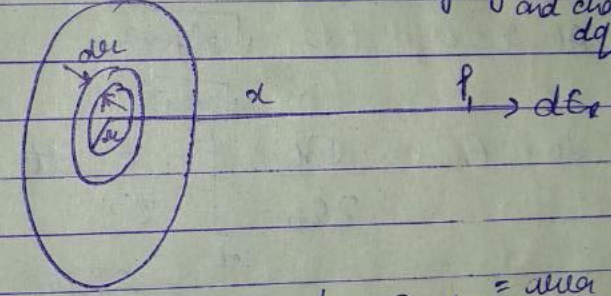Question

# The electric field due to a uniformly charged disc at a point very distant from the surface of the disc is given by [ is the surface charge density on the disc]

A
B
C
D
Medium
SolutionVerified by Toppr
Correct option is A)

## Consider the disc of radius "" with uniform charge density ; be the electric field at a point along the axis of the disc at a distance from its center.We can assume the charge distribution as a collection of concentric rings of charge. Consider one such ring of radius and charge .Area element area of ring charge of that ringDue to symmetry, there is no vertical component of electric field at point P. There is only the horizontal component so, using the result from a ring of charge; put Differentiating both sides, At At So,   is very small compared to 1.So, .00